2.1.2 Calculation of the Jacobian MatrixNext: 2.1.3 Eigenvalues Analysis of Up: 2.1 Nonlinear Least Squares Previous: 2.1.1 Algorithm Description

## 2.1.2 Calculation of the Jacobian Matrix

The calculation of the elements of the jacobian matrix requires the values of the derivatives of the model function at the input data points. TCAD model functions are generally smooth but their analytical derivatives are unavailable. Numerical differentiations techniques are used to   approximate the derivative using forward differences formulae:or a central difference ones:where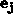is the unit vector in thedirection andrepresents a small increment.

It was proven that the use of numerical approximation in the Levenberg-Marquradt algorithm does not jeopardize its convergence properties . It is also known that numerical differentiation is an unstable procedure prone to truncation and subtractive cancellation errors . Decreasing the step size () will reduce the truncation error. Unfortunately a smaller step has the opposite effect on the cancellation error. Selecting the optimal step size for a certain problem is computationally expensive  and the benefits achieved are not justifiable as the effect of small errors in the values of the elements of the Jacobian matrix is minor. For this reason, the sizing of the finite difference step is not attempted and a constant increment size is used in evaluating the gradient. Furthermore, to allow for the flexibility to adjust to the specific problem characteristics, the individual size of each parameter step can be adjusted by the user.

Calculation of the numerical approximation of the Jacobian matrix requiresmodel evaluations for the forward difference case and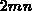for central differences. To alleviate the computational burden, forward differences are always used except when they become unreliable. This usually happens near the solution when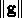is small, or when the difference in the values of the function is small relative to the step size. In both cases, the central difference formulae is used instead. Finally, a first-order Broyden update scheme is implemented and can also be used   to reduce the number of model function computations in the calculation of the approximate Jacobian at the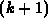iteration as follows: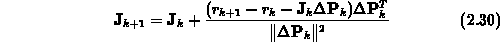This scheme requires onlyfunctional evaluation and can be used up totimes in sequence after each evaluation of the Jacobian matrix by forward or central differences. A note of caution is due here: For highly nonlinear or poorly conditioned models, the errors encountered in using the Broyden update scheme could cause larger parameter deviation which could result in the trapping of the solution at a local minimum.Next: 2.1.3 Eigenvalues Analysis of Up: 2.1 Nonlinear Least Squares Previous: 2.1.1 Algorithm Description

Martin Stiftinger
Tue Aug 1 19:07:20 MET DST 1995